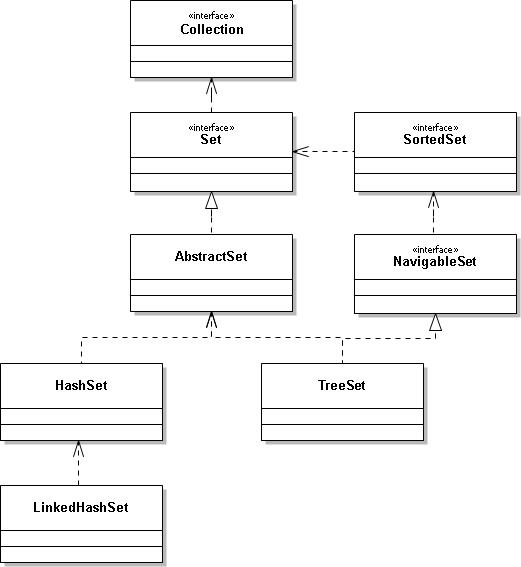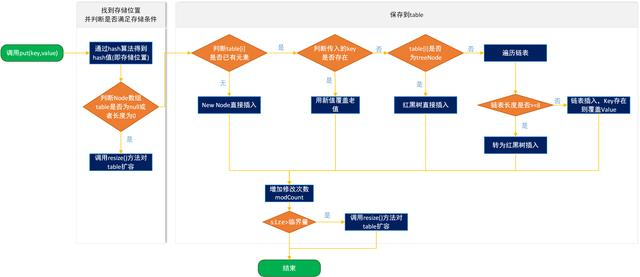# Java高级架构师-Java集合 HashSet的原理及常用方法HashSet是Java集合Set的一个实现类，Set是一个接口，其实现类除HashSet之外，还有TreeSet，并继承了Collection，HashSet集合很常用，同时也是程序员面试时经常会被问到的知识点，下面是结构图public class HashSet<E> extends AbstractSet<E> implements Set<E>, Cloneable, java.io.Serializable{}

HashSet有几个重载的构造方法，我们来看一下

private transient HashMap<E,Object> map;//默认构造器public HashSet() { map = new HashMap<>();}//将传入的集合添加到HashSet的构造器public HashSet(Collection<? extends E> c) { map = new HashMap<>(Math.max((int) (c.size()/.75f) + 1, 16)); addAll(c);}//明确初始容量和装载因子的构造器public HashSet(int initialCapacity, float loadFactor) { map = new HashMap<>(initialCapacity, loadFactor);}//仅明确初始容量的构造器（装载因子默认0.75）public HashSet(int initialCapacity) { map = new HashMap<>(initialCapacity);}

HashMap的数据存储是通过数组+链表/红黑树实现的，存储大概流程是通过hash函数计算在数组中存储的位置，如果该位置已经有值了，判断key是否相同，相同则覆盖，不相同则放到元素对应的链表中，如果链表长度大于8，就转化为红黑树，如果容量不够，则需扩容（注：这只是大致流程）。

HashMap原理(一) 概念和底层架构

HashMap原理(二) 扩容机制及存取原理

private static final Object PRESENT = new Object();public boolean add(E e) { return map.put(e, PRESENT)==null;}HashSet的remove方法通过HashMap的remove方法来实现

//HashSet的remove方法public boolean remove(Object o) { return map.remove(o)==PRESENT;}//map的remove方法public V remove(Object key) { Node<K,V> e; //通过hash(key)找到元素在数组中的位置，再调用removeNode方法删除 return (e = removeNode(hash(key), key, null, false, true)) == null ? null : e.value;}/** * */final Node<K,V> removeNode(int hash, Object key, Object value, boolean matchValue, boolean movable) { Node<K,V>[] tab; Node<K,V> p; int n, index; //步骤1.需要先找到key所对应Node的准确位置，首先通过(n - 1) & hash找到数组对应位置上的第一个node if ((tab = table) != null && (n = tab.length) > 0 && (p = tab[index = (n - 1) & hash]) != null) { Node<K,V> node = null, e; K k; V v; //1.1 如果这个node刚好key值相同，运气好，找到了 if (p.hash == hash && ((k = p.key) == key || (key != null && key.equals(k)))) node = p; /** * 1.2 运气不好，在数组中找到的Node虽然hash相同了，但key值不同，很明显不对， 我们需要遍历继续 * 往下找； */ else if ((e = p.next) != null) { //1.2.1 如果是TreeNode类型，说明HashMap当前是通过数组+红黑树来实现存储的，遍历红黑树找到对应node if (p instanceof TreeNode) node = ((TreeNode<K,V>)p).getTreeNode(hash, key); else { //1.2.2 如果是链表，遍历链表找到对应node do { if (e.hash == hash && ((k = e.key) == key || (key != null && key.equals(k)))) { node = e; break; } p = e; } while ((e = e.next) != null); } } //通过前面的步骤1找到了对应的Node,现在我们就需要删除它了 if (node != null && (!matchValue || (v = node.value) == value || (value != null && value.equals(v)))) { /** * 如果是TreeNode类型，删除方法是通过红黑树节点删除实现的，具体可以参考【TreeMap原理实现 * 及常用方法】 */ if (node instanceof TreeNode) ((TreeNode<K,V>)node).removeTreeNode(this, tab, movable); /** * 如果是链表的情况，当找到的节点就是数组hash位置的第一个元素，那么该元素删除后，直接将数组 * 第一个位置的引用指向链表的下一个即可 */ else if (node == p) tab[index] = node.next; /** * 如果找到的本来就是链表上的节点，也简单，将待删除节点的上一个节点的next指向待删除节点的 * next,隔离开待删除节点即可 */ else p.next = node.next; ++modCount; --size; //删除后可能存在存储结构的调整，可参考【LinkedHashMap如何保证顺序性】中remove方法 afterNodeRemoval(node); return node; } } return null;}

removeTreeNode方法具体实现可参考 TreeMap原理实现及常用方法

HashSet作为集合，有多种遍历方法，如普通for循环，增强for循环，迭代器，我们通过迭代器遍历来看一下

public class LinkedHashSet<E> extends HashSet<E> implements Set<E>, Cloneable, java.io.Serializable { public LinkedHashSet(int initialCapacity, float loadFactor) { super(initialCapacity, loadFactor, true); } public LinkedHashSet(int initialCapacity) { super(initialCapacity, .75f, true); } public LinkedHashSet() { super(16, .75f, true); } public LinkedHashSet(Collection<? extends E> c) { super(Math.max(2*c.size(), 11), .75f, true); addAll(c); } public Spliterator<E> spliterator() { return Spliterators.spliterator(this, Spliterator.DISTINCT | Spliterator.ORDERED); }}

public class TreeSet<E> extends AbstractSet<E> implements NavigableSet<E>, Cloneable, java.io.Serializable{ public TreeSet() { this(new TreeMap<E,Object>()); } public TreeSet(Comparator<? super E> comparator) { this(new TreeMap<>(comparator)); } public TreeSet(Collection<? extends E> c) { this(); addAll(c); } public TreeSet(SortedSet<E> s) { this(s.comparator()); addAll(s); }}

2019-07-28 19:29:37
0 热度